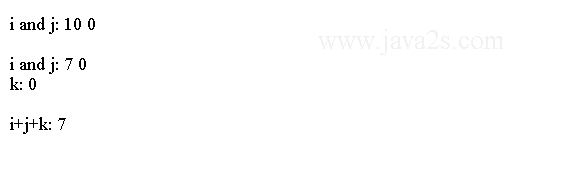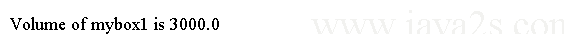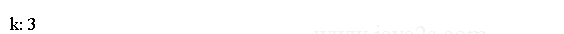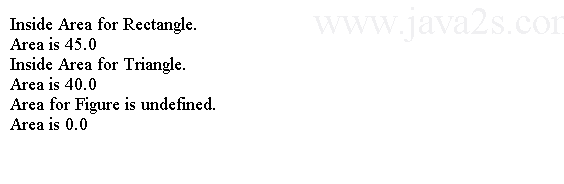# Java Tutorial - Java inheritance

In Java the classes can inherit attributes and behavior from pre-existing classes. The pre-existing classes are called base classes, superclasses, or parent classes. The new classes are known as derived classes, subclasses, or child classes. The relationships of classes through inheritance form a hierarchy.

Let's look at an example. Suppose we have a class called Employee. It defines first name, last name. Then we want to create a class called Programmer. Programmer would have first name and last name as well. Rather than defining the first name and last name again for Programmer we can let Programmer inherit from Employee. In this way the Programmer would have attributes and behavior from Employee.

To inherit a class, you can use the `extends` keyword.

The following program creates a superclass called `Base` and a subclass called `Child`.

```
class Base {/*from   www.  j a va 2s . co  m*/
int i, j;
void showBase() {
System.out.println("i and j: " + i + " " + j);
}
}
class Child extends Base {
int k;
void showChild() {
System.out.println("k: " + k);
}
void sum() {
System.out.println("i+j+k: " + (i + j + k));
}
}

public class Main {
public static void main(String args[]) {
Base superOb = new Base();
Child subOb = new Child();

superOb.i = 10;
superOb.showBase();
System.out.println();

subOb.i = 7;
subOb.showBase();
subOb.showChild();
System.out.println();

subOb.sum();
}
}
```

The output from this program is shown here:The subclass `Child` includes all of the members of its superclass. The general form of a class declaration that inherits a superclass is shown here:

```class subclass-name extends superclass-name {
// body of class
}
```

You can only have one superclass for any subclass. Java does not support the multiple inheritance. A class can be a superclass of itself.

## Java super keyword

You can use `super` in a subclass to refer to its immediate superclass. `super` has two general forms.

1. The first calls the superclass' constructor.
2. The second is used to access a member of the superclass.

## Call superclass constructors

To call a constructor from its superclass:

```super(parameter-list);
```
• `parameter-list` is defined by the constructor in the superclass.
• `super(parameter-list)` must be the first statement executed inside a subclass' constructor.

Here is a demo for how to use super to call constructor from parent class.

```
class Box {/*  w  ww.j  a  v a2s.c  o m*/
private double width;
private double height;
private double depth;

Box(Box ob) { // pass object to constructor
width = ob.width;
height = ob.height;
depth = ob.depth;
}
Box(double w, double h, double d) {
width = w;
height = h;
depth = d;
}
double volume() {
return width * height * depth;
}
}
class BoxWeight extends Box {
double weight; // weight of box
BoxWeight(Box ob) { // pass object to constructor
super(ob);
}
}
public class Main {
public static void main(String args[]) {
Box mybox1 = new Box(10, 20, 15);
BoxWeight myclone = new BoxWeight(mybox1);
double vol;

vol = mybox1.volume();
System.out.println("Volume of mybox1 is " + vol);
}
}
```

This program generates the following output:## Reference members from parent class

We can reference member variable from parent class with super keyword. Its general form is:

```super.member
```

`member` can be either a method or an instance variable.

Let's look at the following code.

```
class Base {/*from  w  w w  .  jav  a2  s .  c  o m*/
int i;
}
class SubClass extends Base {
int i; // this i hides the i in A
SubClass(int a, int b) {
super.i = a; // i in A
i = b; // i in B
}
void show() {
System.out.println("i in superclass: " + super.i);
System.out.println("i in subclass: " + i);
}
}
public class Main {
public static void main(String args[]) {
SubClass subOb = new SubClass(1, 2);
subOb.show();
}
}
```

This program displays the following:## Java Method Overriding

Method Overriding happens when a method in a subclass has the same name and type signature as a method in its superclass.

When an overridden method is called within a subclass, it will refer to the method defined in the subclass.

The method defined by the superclass will be hidden. Consider the following:

```
class Base {//from w w w . jav a  2s.  co  m
int i;
Base(int a) {
i = a;
}
void show() {
System.out.println("i:" + i);
}
}
class SubClass extends Base {
int k;
SubClass(int a, int c) {
super(a);
k = c;
}
void show() {
System.out.println("k: " + k);
}
}
public class Main {
public static void main(String args[]) {
SubClass subOb = new SubClass(1, 3);
subOb.show();
}
}
```

The output produced by this program is shown here:## super and overridden method

To access the superclass version of an overridden function, you can do so by using `super`.

```
class Base {//from www . j a  va  2  s .co  m
int i;

Base(int a) {
i = a;
}

void show() {
System.out.println("i: " + i);
}
}

class SubClass extends Base {
int k;

SubClass(int a, int c) {
super(a);
k = c;
}

void show() {
super.show(); // this calls A's show()
System.out.println("k: " + k);

}
}

public class Main {
public static void main(String[] argv) {
SubClass sub = new SubClass(1, 2);
sub.show();
}
}
```

The following output will be generated if you run the code above:## Method overriding vs method overload

Method overriding occurs when the names and the type signatures of the two methods are identical. If not, the two methods are overloaded. For example, consider this modified version of the preceding example:

```
class Base {//ww  w . ja  va2  s.  c  o  m
int i;
Base(int a) {
i = a;
}
void show() {
System.out.println("i: " + i);
}
}
class SubClass extends Base {
int k;
SubClass(int a, int c) {
super(a);
k = c;
}
void show(String msg) {
System.out.println(msg + k);
}
}
public class Main {
public static void main(String args[]) {
SubClass subOb = new SubClass(1, 2);
subOb.show("This is k: ");
subOb.show();
}
}
```

The output produced by this program is shown here:## Java Constructor in hierarchy

In a class hierarchy, constructors are called in order of derivation, from superclass to subclass.

The following program illustrates when constructors are executed:

```
class A {//w  w w. ja v a 2s .co  m
A() {
System.out.println("Inside A's constructor.");
}
}

// Create a subclass by extending class A.
class B extends A {
B() {
System.out.println("Inside B's constructor.");
}
}

// Create another subclass by extending B.
class C extends B {
C() {
System.out.println("Inside C's constructor.");
}
}

public class Main{
public static void main(String args[]) {
C c = new C();
}
}
```

The output from this program is shown here:## Polymorphism

With polymorphism we can call methods from class hierarchy using a uniform interface. The compiler will determine dynamically which implementation to use.

## Dynamic Method Dispatch

When an overridden method is called through a superclass reference, Java determines which version of that method to execute.

Here is an example that illustrates dynamic method dispatch:

```
class Base {//from   www. j a v a  2 s  .c  o  m
void callme() {
System.out.println("Inside A's callme method");
}
}
class SubClass extends Base {
void callme() {
System.out.println("Inside B's callme method");
}
}
class SubClass2 extends Base {
void callme() {
System.out.println("Inside C's callme method");
}
}
public class Main {
public static void main(String args[]) {
Base a = new Base();
SubClass b = new SubClass();
SubClass2 c = new SubClass2();
Base r;
r = a;
r.callme();
r = b;
r.callme();
r = c;
r.callme();
}
}
```

The output from the program is shown here:## Polymorphism Example

The following example uses the run-time polymorphism. It has three classes. The parent class is `Shape`. `Rectangle` and `Triangle` are both extending the `Shape` class. `Shape` defines a method called `area()` which returns the area of a shape. Both Rectangle and Triangle override `area()` method and provide their own version of implementation. When calling the `area()` method we can call it from the same object reference and Java compiler can figure out which `area()` method to use.

```
class Shape {//from ww w .  j  a  v  a  2s  .co  m
double height;
double width;
Shape(double a, double b) {
height = a;
width = b;
}
double area() {
System.out.println("Area for Figure is undefined.");
return 0;
}
}
class Rectangle extends Shape {
Rectangle(double a, double b) {
super(a, b);
}
// override area for rectangle
double area() {
System.out.println("Inside Area for Rectangle.");
return height * width;
}
}
class Triangle extends Shape {
Triangle(double a, double b) {
super(a, b);
}
// override area for right triangle
double area() {
System.out.println("Inside Area for Triangle.");
return height * width / 2;
}
}
public class Main {
public static void main(String args[]) {
Shape f = new Shape(10, 10);
Rectangle r = new Rectangle(9, 5);
Triangle t = new Triangle(10, 8);

Shape figref;

figref = r;
System.out.println("Area is " + figref.area());

figref = t;
System.out.println("Area is " + figref.area());

figref = f;
System.out.println("Area is " + figref.area());
}
}
```

The output from the program is shown here: